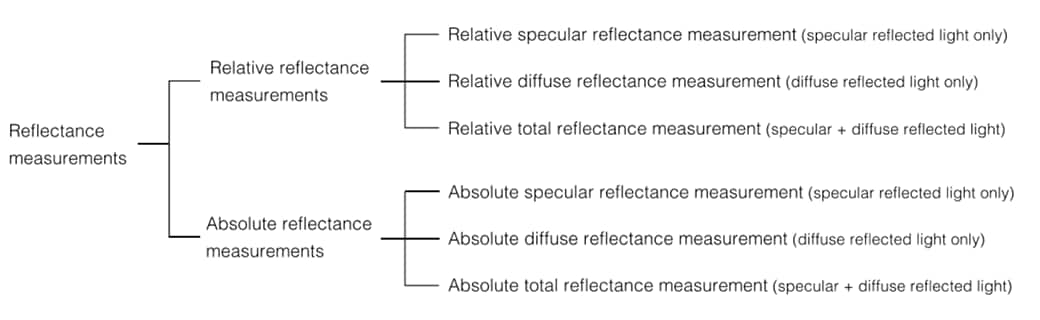# What are the different modes of measuring reflection?Reflectance measurements measure either the relative or absolute reflected light, with measurement values expressed in terms of reflectance.

Relative reflectance measurements calculate the proportional amount of reflected light measured from a sample surface, relative to the amount of reflected light measured from a reference plate, such as barium sulfate or a mirror. The relative reflectance is calculated based on assuming the reference plate has a reflectance of 100 %. Therefore, it is very important to manage reference plates properly because different reflectance values can be obtained if reference plates are substituted or if they become contaminated or change characteristics.

In contrast, absolute reflectance measurements calculate the proportional amount of reflected light relative to the amount of light measured directly from a light source, not using a reference plate such as barium sulfate or a mirror. Reflectance measurement values are based on assuming a 100 % reflectance for air. Absolute reflectance measurements allow for the quantification of the true reflectance of samples, which is referred to as absolute reflectance.

Back to Index